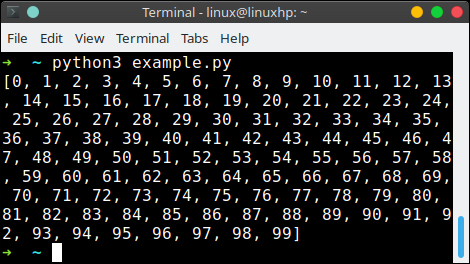What is list comprehension?

List comprehensions are an easy way to create lists.

Its much easier to write a one liner than it is to write a for loop, just for creating a list. This _ one liner_ is called a list comprehension.

Related Course:

## list comprehension example

Assume we want to create a list containing 100 numbers. Manually that would be a lot of typing work. So we would use a for loop, right?

We can define a for loop to fill the list.

We can replace it with a one liners, which is how we obtain the same result:This is also useful if you want to create large lists.

Note: List comprehensions can include function calls and expressions.

Assume we want the square roots to 100: